# Texas Go Math Grade 2 Lesson 16.3 Answer Key Measure in Inches and Feet

Refer to our Texas Go Math Grade 2 Answer Key Pdf to score good marks in the exams. Test yourself by practicing the problems from Texas Go Math Grade 2 Lesson 16.3 Answer Key Measure in Inches and Feet.

## Texas Go Math Grade 2 Lesson 16.3 Answer Key Measure in Inches and Feet

Explore

Draw or write to describe how you did each measurement.
First measurement
Second measurement

FOR THE TEACHER • Have pairs of children stand apart and measure the distance between them with sheets of paper folded in half lengthwise. Then have them measure the same distance using large paper clips.

Math Talk
Mathematical Processes

Describe how the length of a sheet of paper and the length of a paper clip are different.
The length of sheet of paper will be almost 12 inches but the length of the paper clip will be 1 inch.

Model and Draw

12 inches is the same as 1 foot. A 12-inch ruler is about 1 foot long, You can measure lengths in inches and also in feet.
The real table is about 60 inches long. The real table is also about 5 feet long.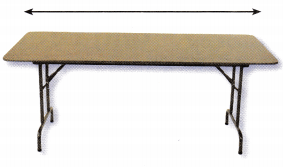Share and Show

Measure to the nearest inch. Then measure to the nearest foot.

Question 1.
Find the real object.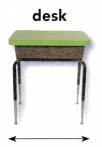Measure
__________ inches
__________ feet
26 inches
2 feet

Explanation:
The length of the desk is about 26 inches and about 2 feet.

Question 2.
Find the real object.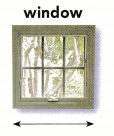Measure
__________ inches
__________ feet
36 inches
3 feet

Explanation:
The length of the window is about 36 inches and about 3 feet.

Question 3.
Find the real object.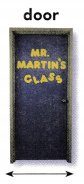Measure
__________ inches
__________ feet
80 inches
6 feet

Explanation:
The length of the door is about 80 inches and about 6 feet.

Problem Solving

Measure to the nearest inch. Then measure to the nearest foot.

Question 5.
Find the real object.Measure
__________ inches
__________ feet
12 inches
1 feet

Explanation:
The length of the poster is about 12 inches and about 1 feet.

Question 6.
Find the real object.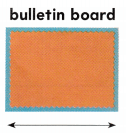Measure
__________ inches
__________ feet
24 inches
2 feet

Explanation:
The length of the bulletin board is about 24 inches and about 2 feet.

Question 6.
H.O.T. Multi-Step Estimate the length of a real shelf in inches and in feet. Then measure.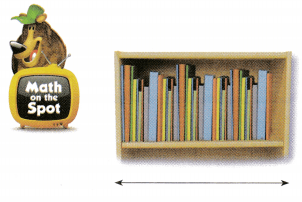Estimates:
__________ inches
__________ feet
Measurements:
__________ inches
__________ feet
Estimates:
30 inches
3 feet

Measurements
35 inches
3 feet

Explanation:
The length of the shelf is about 35 inches and about 3 feet.

Question 7.
H.O.T. Look at your measurements for the shelf. Why is the number of inches different from the number of feet?
The number of inches is different from the number of feet as we know that 1 feet is equal to 12 inches so, the number of feet will be less than number of inches.

Question 8.
Evaluate Reasonableness Joey uses a ruler to measure the length of his pencil box. Which could be the length of his pencil box?
(A) 10 inches
(B) 3 feet
(C) 10 feet
A. 10 inches

Explanation:
Joey uses a ruler to measure the length of his pencil box
A ruler has measurements in inches and centimeters so, the length of the pencil box could be 10 inches.

Question 9.
The length of a poster is 24- inches. Would this same length in feet be more than 24- feet or less than 24- feet? Explain.
The length of the poster is 24 inches, then it could be 2 feet
As we know 12 inches make 1 feet so, 24 inches will be 2 feet
2 feet is less than 24 feet.

Question 10.
Texas Test Prep Stephen is telling his brother about using a ruler. Which sentence is true?
(A) 1 inch is the same length as 1 foot.
(B) 1 foot is a greater length than 1 inch.
(C) 1 inch is a greater length than 1 foot.
(B) 1 foot is a greater length than 1 inch.

Explanation:
As we know that 12 inches make 1 feet then 1 feet will be greater than 1 inch.

TAKE HOME ACTIVITY • Have your child measure a distance in inches and then in feet, and explain why the two measurements are different.
The number of inches is different from the number of feet as we know that 1 feet is equal to 12 inches so, the number of feet will be less than number of inches.

### Texas Go Math Grade 2 Lesson 16.3 Homework and Practice Answer Key

Measure the length to the nearest inch. Then measure to the nearest foot.

Question 1.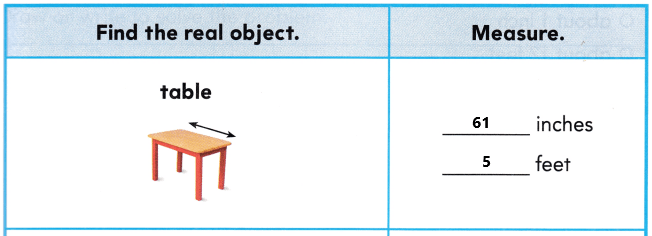Explanation:
The length of the table is about 61 inches and 5 feet long.

Question 2.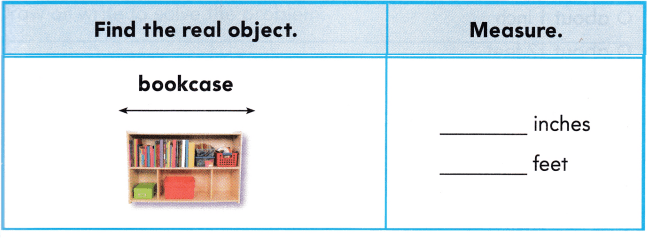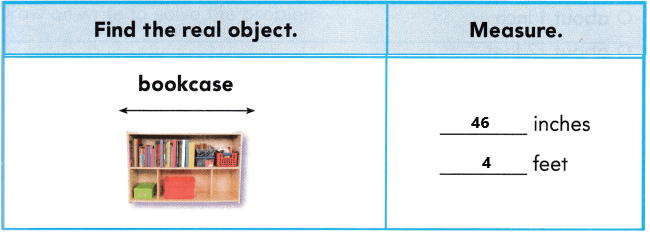Explanation:
The bookcase is about 46 inches and 4 feet long.

Problem Solving

Question 3.
Multi-Step Estimate the length of a real door in inches and in feet. Then measure.
Estimates:
___________ inches
___________ feet
Measurements:
___________ inches
___________ feet
Estimates:
81 inches
7 feet
Measurements:
85 inches
7 feet
Explanation:
The length of the real door is about 85 inches and about 7 feet.

Lesson Check

Question 4.
Angela measures the length of a sheet of paper. She says the paper measures about 11 inches. Which length describes 11 inches?Option A is correct.
Explanation:
Angela measures the length of a sheet of paper. She says the paper measures about 11 inches. The length of the paper is about 1 foot long. So, draw a circle for option A.

Question 5.
Josh uses a ruler to measure the length of his crayon box. Which could be the length of his crayon box?
(A) 5 feet
(B) 5 inches
(C) 1 inchOption B is correct.
Explanation:
Josh used a ruler to measure the length of his crayon box. The length of the crayon box is about 5 inches long. So, draw a circle for option B.

Question 6.
Mr. Scott uses a ruler to measure the width of the classroom door. Which could be the width of the door?
(A) 34 feet
(B) 1 foot
(C) 34 inchesThe width of the classroom door is 34 inches long.
So, option C is correct.
Explanation:
Mr. Scott used a ruler to measure the width of the classroom door. The width of the classroom is 34 inches long. So, draw a circle for option C.

Question 7.
A table is about 60 inches long. How many feet long is the table? (12 inches = 1 foot)
(A) 1 foot
(B) 2 feet
(C) 5 feet